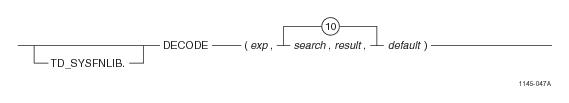# 15.00 - DECODE - Teradata Database

## Teradata Database SQL Functions, Operators, Expressions, and Predicates

Product
Release Number
15.00
Content Type
Programming Reference
Publication ID
B035-1145-015K
Language
English (United States)

## DECODE

### Purpose

Compares expr to each search parameter with its corresponding result parameter.

### Syntaxwhere:

 Syntax element … Specifies … TD_SYSFNLIB the name of the database where the function is located. expr a numeric or character argument. If expr is equal to one of the search arguments, the function returns the corresponding result value. If expr is not equal to any of the search arguments, the functions returns default, if the default value is specified. If expr is NULL, the function returns the result of the first search parameter that is NULL. search a numeric or character argument. DECODE supports 1-10 search arguments tied to an equal number of result arguments. result a numeric or character argument. DECODE supports 1-10 result arguments tied to an equal number of search arguments. default a numeric or character argument. If default is not specified and there are no matches, the function returns NULL.

### ANSI Compliance

This is a Teradata extension to the ANSI SQL:2011 standard.

### Invocation

DECODE is an embedded services system function. For information on activating and invoking embedded services functions, see “Embedded Services System Functions” on page 24.

### Argument Types and Rules

Expressions passed to this function must be one of the following data types:

BYTEINT, SMALLINT, INTEGER, BIGINT, DECIMAL/NUMERIC, FLOAT/REAL/DOUBLE PRECISION, NUMBER, CHAR, VARCHAR

You can also pass arguments with data types that can be converted to the above types using the implicit data type conversion rules that apply to UDFs.

Note: The UDF implicit type conversion rules are more restrictive than the implicit type conversion rules normally used by Teradata Database. If an argument cannot be converted to the required data type following the UDF implicit conversion rules, it must be explicitly cast.

For details, see “Compatible Types” in SQL External Routine Programming.

### Result Type

DECODE is a scalar function whose return value data type depends on the data type associated with the result parameter passed into the function.

• If result or default is a numeric type, DECODE determines which argument has the highest precedence and converts the other result or default arguments to that data type and returns that data type. For details about the order of precedence, see “Compatible Types” in SQL External Routine Programming.
• If that data type is DECIMAL/NUMERIC and the precision and scale of the result or default argument is different, the precision and scale of the return type is set to achieve the maximum precision possible.

For example, if the result / default argument are DECIMAL(6,3), DECIMAL(7,4), and DECIMAL(8,7), the return type would need 3 digits to the left of the decimal point and 7 digits to the right of the decimal point to avoid any reduction in precision. In this case, the return data type is set to DECIMAL(10,7).

In cases where it is not possible to maintain the maximum precision, the data is rounded according to the DBS Control RoundHalfWayMagUp field. For example, if the result and default argument are DECIMAL(32, 8) and DECIMAL(30, 28), the return type is DECIMAL(38,14). This allows for 24 digits to the left of the decimal point required for DECIMAL(32, 8) and 14 digits to the right of the decimal point. If the DECIMAL(30,28) result or default argument is the greatest value, it is rounded to 14 places to the right of the decimal point.

If the data type is fixed point NUMBER and the precision is less than or equal to 38, the precision and scale of the return type are calculated with the same method used for DECIMAL/NUMERIC. However, if the precision is greater than 38, the return type is changed to NUMBER(*) to avoid loss of accuracy. If the data type is floating point NUMBER, the return type is NUMBER(*).

• If result or default is a character data type, the function returns a VARCHAR in the character set of the first result argument.
• For information on the default data type format for DOUBLE PRECISION, see SQL Data Types and Literals.

### Example

The following query:

`SELECT DECODE(country_id, 1, 'United States',`
`                          2, 'England',`
`                          3, 'France',`
`                          'United States')`
`    FROM customers; `

returns:

• 'United States' if the country_id is 1
• 'England' if the country_id is 2
• 'France' if the country_id is 3
• 'United States' if the country_id is not equal to 1, 2, or 3
• ### Example

The following query

`SELECT DECODE(country_id, 1, 'United States', `
`                          2, 'England')`
`    FROM customers; `

returns:

• 'United States' if the country_id is 1
• 'England' if country_id is 2
• NULL if country_id isn't in the range 1 to 2
• ### Example

The following query:

`SELECT DECODE(country_id, 1, 'United States',`
`                          2, 'England',`
`                          NULL, 'France')`
`    FROM customers;`

returns:

• 'United States' if the country_id is 1
• 'England' if the country_id is 2
• NULL if the country_id is NULL
• NULL if the country_id is not equal to 1, 2, or NULL